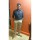Java Developers
Group for Java developers and programmers. Ask questions and get answers related to Java programming
255 Members
Join this group to post and comment.DHIRAJ LOTLIKAR
Information Technology
08 Feb 2019

# What is isNaN() and IsInfinite() methods in java?

isNaN() and isInfinite() methods are the static members of java.lang.Double and java.lang.Float classes. Both these two wrapper classes have their own version of these two methods.

Before discussing about these two methods, let’s get to know about NaN, POSITIVE_INFINITY and NEGATIVE_INFINITY.

NaN, POSITIVE_INFINITY and NEGATIVE_INFINITY are static and final constants of java.lang.Double and java.lang.Float wrapper classes.

NaN : This constant holds Not-a-Number value of specified type. It represents mathematically undefined or unrepresentable number such as number obtained by dividing zero by zero or square root of a negative number.

POSITIVE_INFINITY : This constant holds positive infinity value.

NEGATIVE_INFINITY : This constant holds negative infinity value.